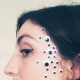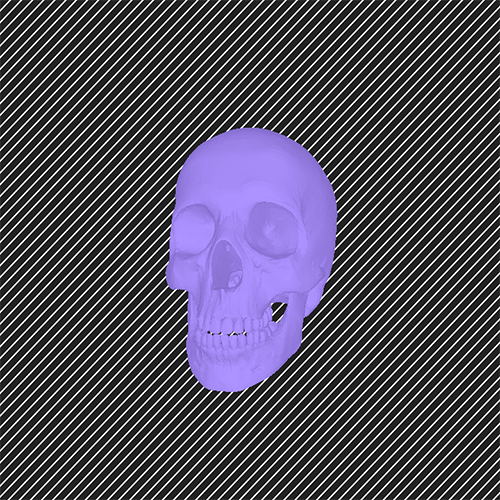# rlciavar

## 10 Feb 2015I created a gif using a 3D file loader addon in openFrameworks. I uploaded a skull .stl file that rotates back and forth about 26 degrees in a non-linear motion. In the background I drew a bunch of diagonal lines that scroll across the screen. I didn’t realize how visually overwhelming this effect would be, but I kind of like it. It was pretty difficult getting a handle of the 3d addon at first, but I was eventually able to work it out. Unfortunately the GIF does not loop; this was an exporting error. Reload this page to see the skull shake its head.A bit of code:

```#include "ofApp.h"

//--------------------------------------------------------------
void testApp::setup(){

ofBackgroundHex(0x222222);
ofSetVerticalSync(true);
glEnable(GL_DEPTH_TEST);
light.enable();

// Move off default colors
light.setDiffuseColor(ofColor::fromHsb(180, 70, 75));
light.setAmbientColor(ofColor::fromHsb(180, 150, 200));

ofSetLogLevel(OF_LOG_VERBOSE);
bSaveModel = false;

drawMode = facets;
}

//--------------------------------------------------------------

void testApp::update(){
if(bSaveModel){
bSaveModel = false;
vector& facets = stlImporter.getFacets();
int numFacets = facets.size();
stlExporter.beginModel("some model");
for(int i=0; i<numFacets; i++){
}

stlExporter.useASCIIFormat(true); //export as ASCII (default is binary)
stlExporter.saveModel(ofToDataPath("testASCII.stl"));
stlExporter.useASCIIFormat(false); //export as binary
stlExporter.saveModel(ofToDataPath("testBin.stl"));
}
}

//--------------------------------------------------------------
void testApp::draw(){
// Enlarge our model
float rachelScale = ofGetWidth()/720.0;
stlImporter.rescaleModel(350 * rachelScale);

//ofLog() << "hi there" << 1;
int nFramesIWant = 10;
float rotationRange = 30;
int whichFrame = ofGetFrameNum() % nFramesIWant;
printf ("Which frame I'm on = %d\n", whichFrame);
float t = ofMap (whichFrame, 0,9, 0,TWO_PI);
float cost = cos(t); // 0...1
float amountToRotateSomething = rotationRange/2.0 * cost;
//float amountToRotateSomething = ofMap (whichFrame, 0,9, 0-rotationRange/2, rotationRange/2);

ofPushMatrix();
ofTranslate (ofGetWidth()/2, ofGetHeight()/2,0);

//red X axis
// ofSetColor (255,0,0);
// ofLine(0,0,0, 1000,0,0);

//green Y axis
// ofSetColor (0,255,0);
// ofLine(0,0,0, 0,1000,0);

ofRotateY ( amountToRotateSomething );
ofRotateX ( 70);
ofRotateZ (-90);
ofTranslate (0,0, 0-100*rachelScale);

ofSetColor (200,200,200);
if(drawMode == normals) {
stlImporter.drawNormals();
}
else if(drawMode == wire) {
stlImporter.drawWireFrame();
}
else if(drawMode == facets) {
stlImporter.drawFacets();
}

ofPopMatrix();
// ofSetHexColor(0xe0d6ff);

//diagonal stripes
ofPushMatrix();
ofScale(1.8*rachelScale,1.8*rachelScale);
ofTranslate (-29*rachelScale,-29*rachelScale,-80*rachelScale);

//finding the edge of the screen
//red X axis for diagonal lines

ofSetColor (255,0,0);
ofLine(0,1,0, 1000,0,0);
//green Y axis for diagonal lines
ofSetColor (0,255,0);
ofLine(1,0,0, 0,1000,0);

//initializing x,y arrays for diagonal lines
const int length = (720+50);
float xPos [length] = {};
float yPos [length] = {};

//populating arrays
for ( int i = 0; i < length; i++) {
xPos[i] += 10*i-50;
}

for (int j = 0; j < length; j++) {
yPos[j] += 10*j-50;
}

ofDisableLighting();
//drawing diagonal lines
ofSetColor(255);
glLineWidth(3);

int moveAmount = 0;